Molar Mass Calculation Exam PracticeUse the bond enthalpies given to estimate the heat released when ethene, CH2=CH2, reacts with HBr to form CH3CH2Br. Bond enthalpies (kJxmołc-H, 412; C-C, 348; C=C, 612; c-Br, 276; Br-Br,193: H-Br, 366.424 I1036 kJ/mol470 kJ/mol200 kJ/molctAnswega kJ/molwered stion 3 o / 5 pts

 Assuming all degrees of motion are excited under the high temperature conditions present, what is the maximum possible molar heat capacity at constant volume for the molecule BBr3?ctAnsweț-Ŕ2.5 R8.5 R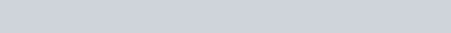Calculate the standard reaction enthalpy for the reaction N2H4(l) + H2(g) —9 2NH3(g) Given: N2(g) + 2 H 20(g) N2H4(l)02(g) = 543 kJ/moł-1H2(g) + % 02(g) H20(g)-1= -242 kJ/mol2 N H3(g) N2(g) + 3 H2(g)AHO = 92.2 kJ/moł-1ctAnswer 51 kJ/mol-935 kJ/mol-243 kJ/mol-1119 kJ/mol -59 kJ/mol
 ered

#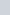stion 5 O / 5 pts

Based on the phase diagram shown, which of the following statements are correct?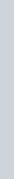c— for—rave —..>

1. Sublimation occurs anywhere along the curve connecting point B to point D.
2. AHfus can be measured at point F.
3. Point E represents a condition where the solid and liquid vapor pressures are the same.
4. At point F the number of independent variables (degrees of freedom) when the liquid and gas are in equilibrium is two.
5. The temperature at point D is called the critical temperature.

Answers’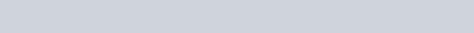Consider the following four molecules 1-4, all with molecular formula C6H14:CH3CHaCH2CHCH3Which has the lowest vapor pressure?4Answere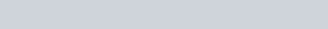23

reectThe combustion of 5.00 grams of C2H6(g), at constant pressure releases 259 kJ of heat. What is AH for the reaction: 2 C2H6(g) + 7 02(g) —s 4 C02(g) + 6 H20(l)?-518 10-1295 kJ-1558 10-259 10rrectAnsw%115 kJ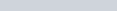Estimate the enthalpy of vaporization of CF2CBr2 given that at 370 C and 870C its vapor pressure is 167 and 585 Torr, respectively. Assume that the enthalpy of vaporization is independent of the temperature.18.6 kJ/moltAnswerzå.3 kJ/mol33.1 kJ/mol -33.1 J/mol-23.3 J/mol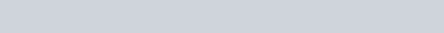How much heat in kJ is required to convert 55.0 g of ice at 0.0 o c into steam (vapor) at 150 oc? Note, the specific heat capacity of ice, water and steam are 2.1, 4.18, and 1.8 J/0C-g, respectively, and AHfus = 6.01 kJ/mol and AHvap = 40 7 kJ/mol for water.16615246.328.0Answerl)l

tt Consider the following reaction: 2 C8H18(l) + 25 02(g) —9 16 C02(g) + 18 H20(l), AHO = -11,20010Standard enthalpies of formation, AHOf: C02(g),-393.5 kJ/mol; H20(l), -285.8 kJ/mol Use the above data to calculate the standard enthalpy of formation of octane, C8H18(l).-240 kJ/mol -6296 kJ/molAnswerO kJ/mol-11,440 kJ/mol-5144 kJ/mol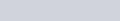11

 For each of the following groups of substances, pick the one that has the given property for each of the items (i), (ii), and (iii), respectively.Highest boiling point: CH4, CF4, H20.Greatest heat of vaporization: HCI, HBr, HI. (iii) Lowest melting point: NaCl, N2, CHC13.CF4, HBr, CHC13Answer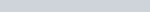-mao, HI, N21-120, HCI, NaCl1-420, HI, NaClCH4, HCI, N2
 swered

stion 12 0/ 5 pts

 Which of the following would be expected to be characteristic of n-type silicon?Electrons are the mobile charge carriers.The electrical conductivity increases with increasing temperature.The dopant could be Boron.The dopant could be Phosphorus.Holes are the mobile charge carriers.1, 2 3Ahswerxectn swered

stion 14 0/ 5 pts

 A gas is allowed to expand at constant temperature from a volume of 2.0 L to 11.2 L against an external pressure of 0.750 atm. If the gas absorbs 128 J of heat from the surroundings, what are the values of q, w, and AE, respectively?128 J, 699 J, 827 J -128 J, -6.9 J, -135 J 128 J, -6.9 J, 121 J128 J, 6.9 J, 135 JAnswerx28 J, -699 J, -571 J

rect

stion 15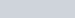A hydrocarbon sample was burned in a bomb calorimeter. The temperature of the calorimeter and the 1.00 kg of water rose from 20.45 0C to23.65 0C. The heat capacity of the calorimeter, excluding the water, is 2.21 kJ/0C. Using this information, determine the heat of this combustion reaction. The specific heat capacity of liquid water is 4.186 J/g- 0C.-86.8 k]swerzo 5 kJ-46.0 10-10.3 10-13.3k]

Unanswered stion 16 0/ 5 pts

 r How much heat must be supplied to a 100 g glass jar containing 20 g of water to raise the temperature from 25 0 C to 50’C? The specific heat capacities of glass and water are 0.78 and 4.18J g C , respectively (assume no heat is lost to the surroundings).14.9 k]2.09 k]10.8 k]Answe&ö4 kJ1.95 k]

stion 17 0/ 5 pts

 Correct Calculate the vapor pressure in torr at 25’C of a solution containing 26.9 g of the nonvolatile, nonelectrolyte (i.e. non-dissociating) solute: urea, CO(NH2)2, in 712 g H20. The vapor pressure of water at 25 0 C is 23.8 torr.0.2710.70.6022.9Answer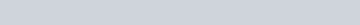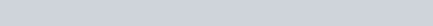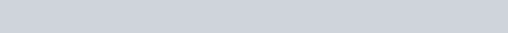orrect Calculate the total vapor pressure at 25 0C of a mixture of benzene and toluene in which the mole fraction of benzene is 0.650. The vapor pressure at25 0C of benzene is 94.6 Torr and that of toluene is29.1 Torr.84.4 Torr51.3 Torr61.5 Torr124 TorrAnswe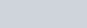.7Calculate the solubility (in moles/liter) of N2 in water at a partial pressure of N2 of 435 torr at 200C. The Henry’s law constant for N2 in water at200C is 7.0 x 10- mol / liter -atm.-21.0 x 1053.0 x 10-13.0 x 10-4 2.1 x 10swes.o x 10 4stion 21

 The metal titanium, Ti, has a body centered cubic3 unit cell. The density of titanium is 4.50 g/cm Calculate the edge length (i.e. the lattice parametera) for the unit cell, in angstroms (IÅ = 10- cm).2.60 Å6.71Å5.32 ÅAnswe&28 Å4.13

Correct

stion 22 0/ 5 pts

 Which of the following solutes dissolved in 1.0 kg of water would be expected to boil at the highest temperature? Assume all of the salts fully dissociate to ions.0.045 moles of calcium sulfite, CaS03.0.025 moles of sodium selenide, Na2Se.0.025 moles of ferric (iron Ill) chloride, FeC13.0.040 moles of rubidium chloride, RbCl. rrectAnswer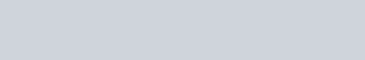Co

stion 23 0/ 5 pts

 A 0.750 molal aqueous solution of lithium fluoride (LiF) freezes at -1.95 0 C. Given this information, what is the value for the van’t Hoff factor i which describes the relationship between the moles of particles in solution compared to the moles of solute dissolved? Kf = 1.86 0 C kg/mole for H20.2.8 tAnswe%40.81.92.3

Correc

stion 24

 The molecular mass of hemoglobin (with i = 1) is 6.86 x 10 g/mol. What mass of hemoglobinmust be present in 150.0 mL of solution to exert an osmotic pressure of 132.2 torr at 25″C?Answera.2 g23.0 g5.56 kg37.6 g87.2 g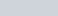t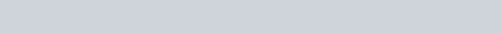Correct Which of the following solutes is likely to be most soluble in water?Ghanol, CH3CH20Hcarbon tetrachloride, CC14 sulfur hexabromide, SBr6Br2CS2t The vapor pressure of water at 7(YC is 365 torr. What is the vapor pressure (in torr) of an ideal solution made by dissolving 0.90 moles of glucose(C6H1206) in 144 g of water?Answe&28373632443.2

Cor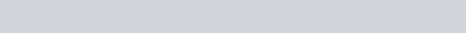What is the boiling point for a solution containing 40.2 g of anthracene (a nonvolatile, nondissociating hydrocarbon molecule of formula C14H10) in 285. g of liquid benzene? (Note: the normal boiling point of benzene, C6H6, is 80.100 C, and Kb = 2.53 OC/m for benzene).Answer .100c85.oooc78.100c83.420c80.460C

rect

 n swered

stion 28 0/ 5 pts

 The vapor pressure of pure benzene (C6H6) at 25 0C is 94.4 torr while the vapor pressure of pure chloroform (CHC13) at 25 0C is 172 torr. An ideal solution is formed when 50 g of chloroform is added to 50 g of benzene. Calculate the vapor pressure of chloroform over this chloroformbenzene solution at 250 C.172 torrAnswegg torr148 torr125 torr86 torr
 n swered

stion 29 0/ 5 pts

 rect Silver chloride, AgCl, crystallizes with the rock salt (NaCl) ionic crystal structure. The length of the unit cell edge (i.e. the lattice parameter a) is 5.55 angstroms (1 angstrom = 10- cm). What is the density of AgCl, in g/cm ?4.1966.1 x 10Answer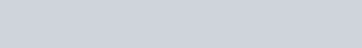Hydrogen peroxide (1-4202) is a powerful oxidizing agent used in concentrated solution in rocket fuels and in dilute solution as a hair bleach. An aqueous solution of H202 is 30.0 % by mass and has a density of 1.11 g/mL. Calculate its molarity (in mol/L).0.0138.812.6300An web-79
 okrect The addition of 125 mg of caffeine to 100 g of cyclohexane lowered the freezing point by 0.13 K. Calculate the molar mass of caffeine. The Kf for cyclohexane is 20.1 Kxkgxmol-481 g/mol96.5 g/mol19.3 g/molAnswe%93 g/mol47.8 g/mol